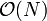# Difference between revisions of "Queue"

A queue is a data structure containing zero or more items, all of the same type, which supports two basic operations:

• Push an element onto the queue.
• Pop the first value off the queue, returning its value in the process.

In practice, we might choose to include the following operations as well:

• Construct an empty queue.
• Copy the contents of one queue to another.
• Peek at the first element in the queue (the one which would be popped next), without removing it.
• Empty an already existing queue.
• Find size of a queue.
• Test if empty.

Unlike with a stack, a peek operation cannot be implemented on a queue as a pop followed by a push. (The test if empty operation is still the same as the find size operation followed by a test of whether or not the size is zero.)

# Terminology

The term queue is based off of real-life queues; they are often referred to as lines, as in the case of a queue for a ride in an amusement park. In a queue like this, we join the end of the queue (become last) and wait until we are at the front (become first) before we are popped (let in). In computer science, the most recently pushed element is last, whereas the least recently pushed element is first. An element can be described as before another if the latter was pushed later; the latter is referred to as being after the former. To push means to add an element, and to pop means to remove an element.

# Implementation

### Array implementation

In an array implementation of a queue, the contents of the queue are stored in consecutive indices in an array. However, we encounter a problem if we want the element in the lowest-indexed position (i.e. 0 or 1) to be constantly at the front: when we pop, we have to shift over all the other elements so that the lowest-indexed position contains the next element to be popped and all elements remain contiguous. This can take$\mathcal{O}(N)$ time where$N$ is the number of elements currently in the queue. But we need constant time push/pop operations in order to make important algorithms like BFS run in linear time. To solve this, we do not replace the popped element, we merely increment an index into the next element to be popped. That is, we maintain two indices into the array: one to the front and one to the back. When we push, we add an element to the back and increment the back index; when we pop, we remove the element from the front and increment the front index. However, consider what happens if, for example, we continually push one element, then pop one, then push another, and pop another, and so on: the size of the queue doesn't change much, but both indices will eventually go out of range. To fix this problem, we allow the indices to wrap around, so that incrementing the highest possible index will give the lowest possible one. This is done using the modulo operation. Following is an exemplary implementation:

object queue
function construct(max_size)
let A be an array that can hold at least max_size+1 elements
let N = max_size+1
let first = 0
let last = 0
function push(x)
A[last] = x
last = (last + 1) mod N
function pop
return_val = A[first]
first = (first + 1) mod N
return return_val
function peek
return A[first]
function size
return (last-first) mod N
function make_empty
last = first


Notice that if the queue becomes full and we attempt to push more elements, then the apparent size will wrap to zero and the earliest-pushed elements will be overwritten. Also, if we attempt to pop an empty queue, the apparent size will wrap to the largest possible value. Error-checking code is fairly simple to add but, like the test if empty and copy operations, it has been omitted for the sake of brevity.